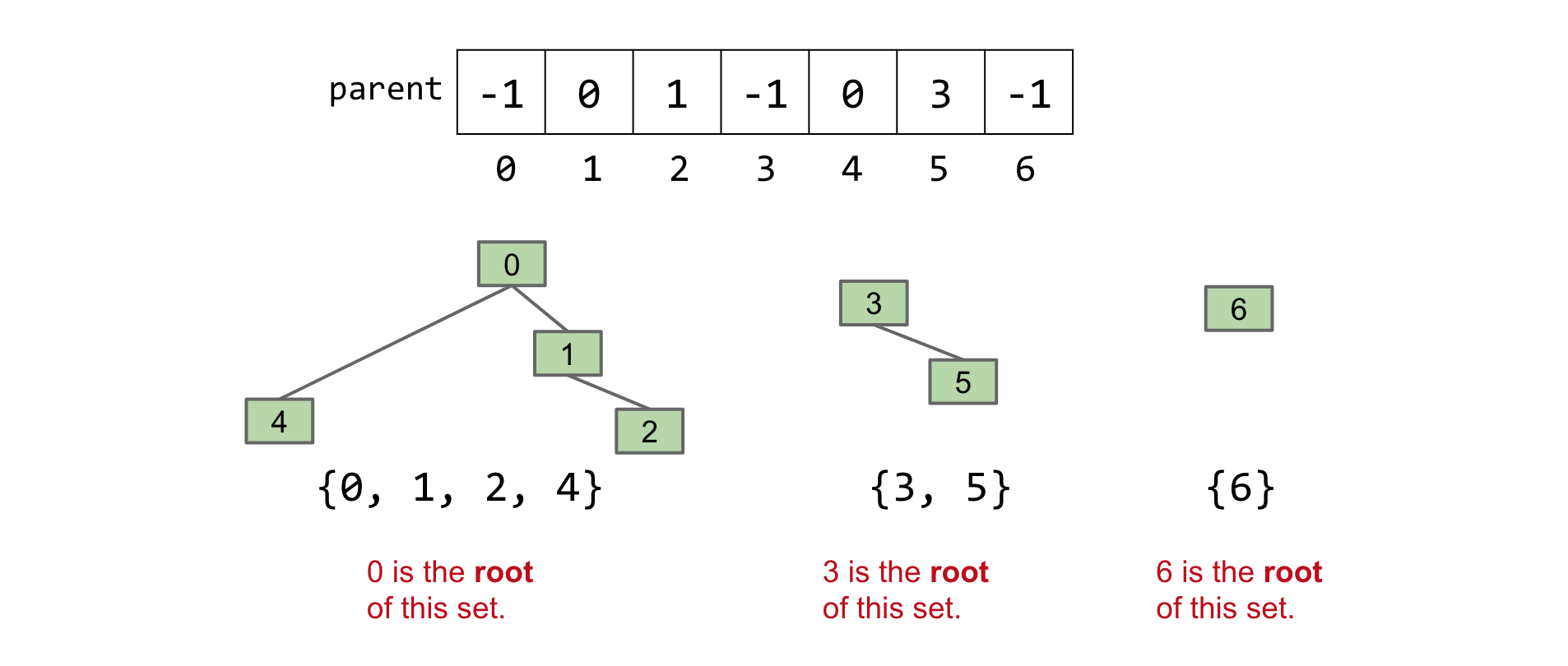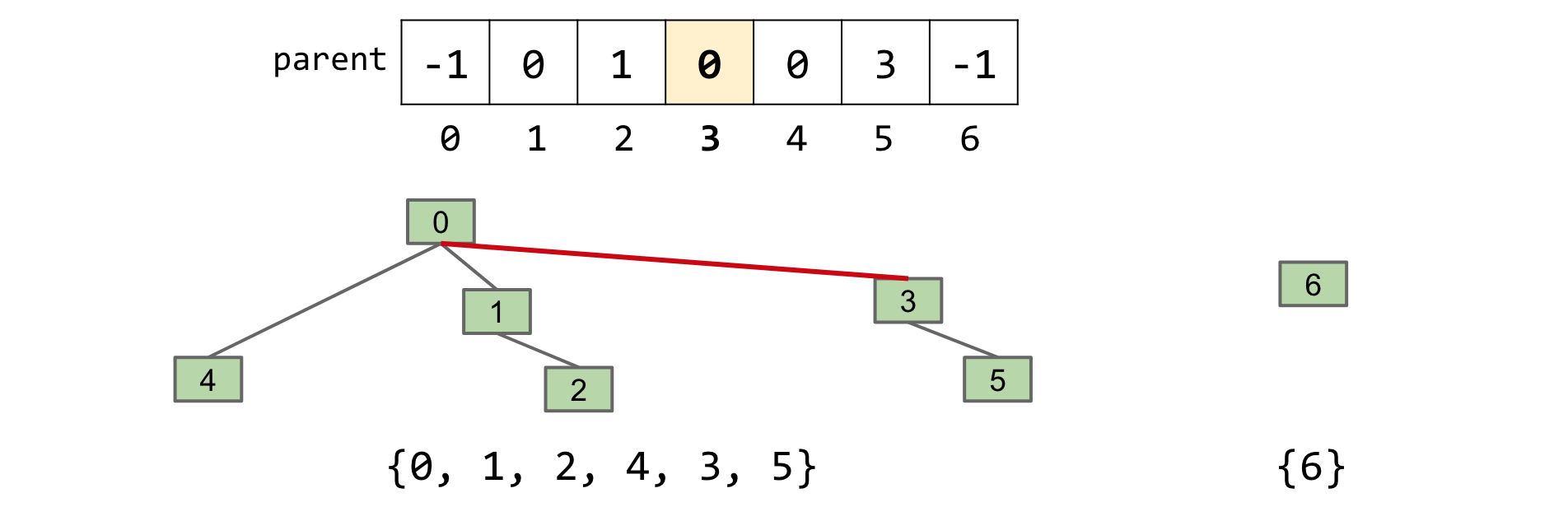## Quick Union

Suppose we prioritize making the connect operation fast. We will still represent our sets with an array. Instead of an id, we assign each item the index of its parent. If an item has no parent, then it is a 'root' and we assign it a negative value.

This approach allows us to imagine each of our sets as a tree. For example, we represent {0, 1, 2, 4}, {3, 5}, {6} as:Note that we represent the sets using only an array. We visualize it ourselves as trees.

For QuickUnion we define a helper function find(int item) which returns the root of the tree item is in. For example, for the sets above, find(4) == 0, find(1) == 0, find(5) == 3, etc. Each element has a unique root.

#### connect(x, y)

To connect two items, we find the set that each item belongs to (the roots of their respective trees), and make one the child of the other. Example:

connect(5, 2):

1. find(5) -> 3
2. find(2) -> 0
3. Set find(5)'s value to find(2) aka parent = 0Note how element 3 now points to element 0, combining the two trees/sets into one.

In the best case, if x and y are both roots of their trees, then connect(x, y) just makes x point to y, a Θ(1) operation! (Hence the name QuickUnion)

#### isConnected(x, y)

If two elements are part of the same set, then they will be in the same tree. Thus, they will have the same root. So for isConnected(x, y) we simply check if find(x) == find(y).

### Performance

There is a potential performance issue with QuickUnion: the tree can become very long. In this case, finding the root of an item (find(item)) becomes very expensive. Consider the tree below:In the worst case, we have to traverse all the items to get to the root, which is a Θ(N) runtime. Since we have to call find for both connect and isConnected, the runtime for both is upper bounded by O(N).

### Summary and Code

Implementation Constructor connect isConnected
QuickUnion Θ(N) O(N) O(N)
QuickFind Θ(N) Θ(N) Θ(1)
QuickUnion Θ(N) O(N) O(N)

N = number of elements in our DisjointSets data structure

From the runtime chart, QuickUnion seems worse than QuickFind! Note however that O(N) as an upper bound. When our trees are balanced, both connect and isConnected perform reasonably well. In the next section we'll see how to guarantee they perform well.

public class QuickUnionDS implements DisjointSets {
private int[] parent;

public QuickUnionDS(int num) {
parent = new int[num];
for (int i = 0; i < num; i++) {
parent[i] = i;
}
}

private int find(int p) {
while (parent[p] >= 0) {
p = parent[p];
}
return p;
}

@Override
public void connect(int p, int q) {
int i = find(p);
int j= find(q);
parent[i] = j;
}

@Override
public boolean isConnected(int p, int q) {
return find(p) == find(q);
}
}